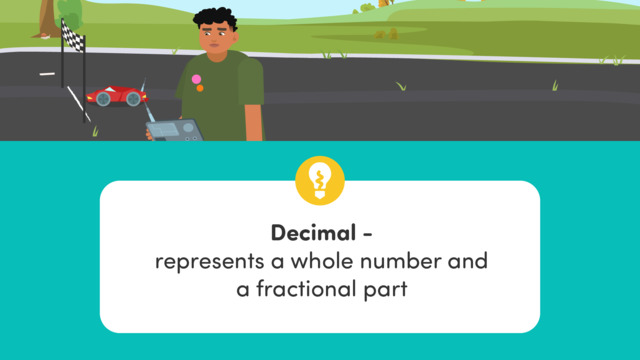# Subtracting Decimals using Place ValueRating

Ø 4.5 / 4 ratings
The authorsTeam Digital
Subtracting Decimals using Place Value
CCSS.MATH.CONTENT.5.NBT.B.7

## Basics on the topicSubtracting Decimals using Place Value

How do you subtract decimal numbers? Learn how to subtract decimals with this video!

### TranscriptSubtracting Decimals using Place Value

Luis has finished his latest project: a mini electric race car! The distance travelled must be monitored to make sure it doesn't run out of battery. To do this, Luis can use subtracting decimals using place value. A decimal is a way of representing a whole number and a fractional part. Our knowledge of place value can help us when subtracting decimals. Here is the decimal point, and the values to the right represent the fractional parts; the tenths and hundredths. So, how do we subtract decimals? Let's use the example five and two tenths minus three and fifteen hundredths. First, align the numbers by place value. Then, subtract just like you would with whole numbers, starting from the rightmost place value. With decimal values, you will sometimes find that there is no digit in a place value, like here in the hundredths column. When this happens, always assume it to be zero, and write a zero as a placeholder. This ensures your calculations are accurate. In the hundredths column, we cannot subtract five from zero, so take one from column to the left. Now the hundredths place is ten, so ten minus five is five. Now we have a one in the tenth's column and when we subtract we have zero left. Remember to add the decimal place before we move on. Now in the whole numbers, we continue subtracting in the ones column and get two. Five and two tenths subtract three and fifteen hundredths is two and five hundredths. Let's help calculate the range left in Luis' electric car after each turn. It starts with a range of twenty five and thirty three hundredths feet. Luis will first drive it for five and two hundredths feet. How much range is left? Pause the video to work on the problem, and press play when you are ready to see the solution. First, you align the place values. In the hundredths place, three minus two is one. In the tenths place, three minus zero is three. Remember, always add the decimal point in the correct place. In the ones place, five subtract five is zero, and two subtract zero is two in the tens place. Luis' car still has a range of twenty and one hundredth feet. Next, Luis drives the electric race car for eighteen and two tenths feet. How much range is left? Pause the video to work on the problem, and press play when you are ready to see the solution. First, you align the place values. Add a zero here as a placeholder. In the hundredths place, one minus zero is one. We need to regroup in the tenths place since two cannot be taken from zero, and since there is a zero in the ones place as well, we'll need to start our regrouping from the tens place. Ten minus two is eight. Remember to always add the decimal point. In the ones place, nine minus eight is one, and in the tens place, one minus one is zero. Luis' car has a range of one and eighty-one hundredths feet left. While Luis continues driving his electric car, let's wrap it up. To summarize, when subtracting decimals, always align the place values, and solve from right to left. Always make sure the decimal point is placed in the correct column. Subtracting decimals is a useful skill when it comes to calculating differences in money, measurements, or distance. It looks like Luis' final problem is getting back to the charging dock!